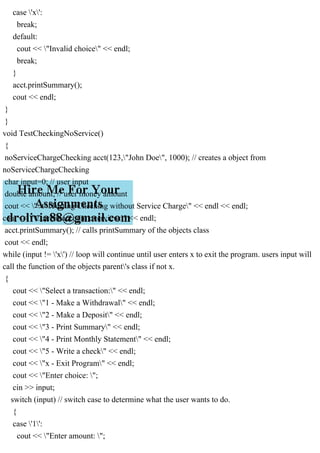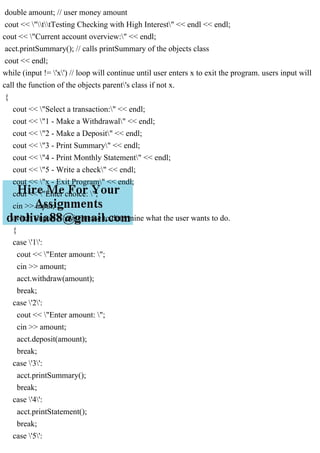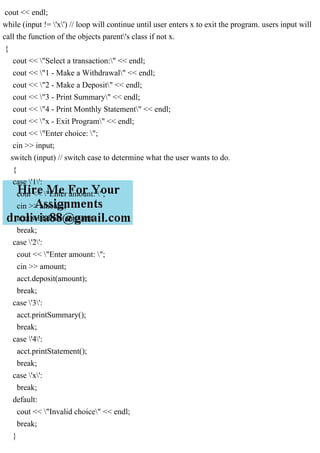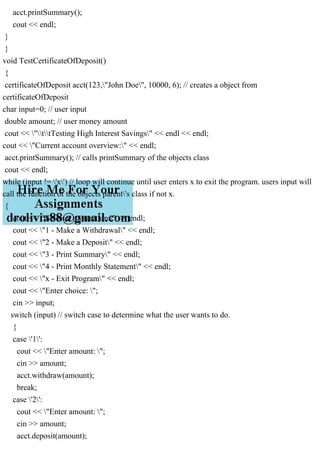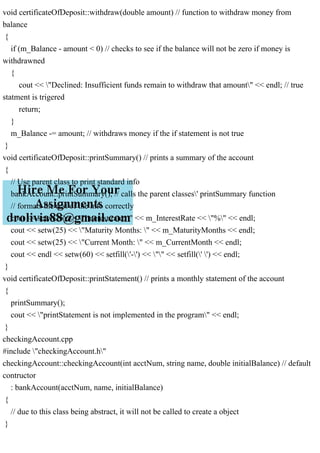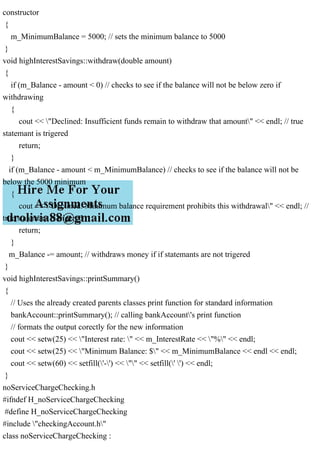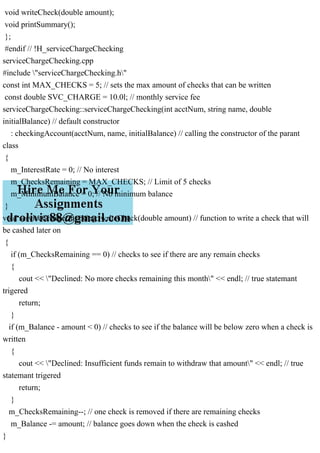Próximo SlideShare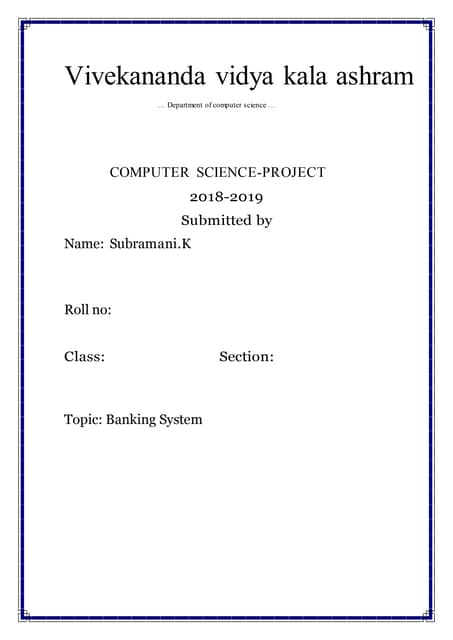Cbsecomputersciencecclass12boardproject bankmanagmentsystem-180703065625-conv...
Cargando en ... 3
1 de 24

### Banks offer various types of accounts, such as savings, checking, cer.pdf

1. Banks offer various types of accounts, such as savings, checking, certificate of deposits, and money market, to attract customers as well as meet their specific needs. Two of the most commonly used accounts are savings and checking. Each of these accounts has various options. For example, you may have a savings account that requires no minimum balance but has a lower interest rate. Similarly, you may have a checking account that limits the number of checks you may write. Another type of account that is used to save money for the long term is certificate of deposit (CD). In this programming exercise, you use abstract classes and pure virtual functions to design classes to manipulate various types of accounts. For simplicity, assume that the bank offers three types of accounts: savings, checking, and certificate of deposit, as described next. Savings accounts: Suppose that the bank offers two types of savings accounts: one that has no minimum balance and a lower interest rate and another that requires a minimum balance and has a higher interest rate. Checking accounts: Suppose that the bank offers three types of checking accounts: one with a monthly service charge, limited check writing, no minimum balance, and no interest; another with no monthly service charge, a minimum balance requirement, unlimited check writing and lower interest; and a third with no monthly service charge, a higher minimum requirement, a higher interest rate, and unlimited check writing. Certificate of deposit (CD): In an account of this type, money is left for some time, and these accounts draw higher interest rates than savings or checking accounts. Suppose that you purchase a CD for six months. Then we say that the CD will mature in six months. The penalty for early withdrawal is stiff. bankAccount: Every bank account has an account number, the name of the owner, and a balance. Therefore, instance variables such as name, accountNumber, and balance should be declared in the abstract class bankAccount. Some operations common to all types of accounts are retrieve account owner's name, account number, and account balance; make deposits; withdraw money; and create monthly statements. So include functions to implement these operations. Some of these functions will be pure virtual. checkingAccount: A checking account is a bank account Therefore, it inherits all the properties of a bank account. Because one of the objectives of a checking account is to be able to write checks, include the pure virtual function writeCheck to write a check. serviceChargeChecking: A service charge checking account is a checking account. Therefore, it inherits all the properties of a checking account. For simplicity, assume that this type of account does not pay any interest, allows the account holder to write a limited number of checks each month, and does not require any minimum balance. Include appropriate named constants, instance variables, and functions in this class. noServiceChargeChecking: A checking account with no monthly service charge is a checking account. Therefore, it inherits all the properties of a checking account. Furthermore, this type of account pays interest, allows the account holder to write checks, and requires a minimum balance. highlnterestChecking: A checking account with high interest is a checking account with nomonthly service charge.
2. Therefore, it inherits all the properties of a no service charge checking account. Furthermore, this type of account pays higher interest and requires a higher minimum balance than the no service charge checking account savingsAccount: A savings account is a bank account. Therefore, it inherits all the properties of a bank account. Furthermore, a savings account also pays interest. highlnterestSavings: A high-interest savings account is a savings account. Therefore, it inherits all the properties of a savings account. It also requires a minimum balance. certificateOfDeposit: A certificate of deposit account is a bank account. Therefore, it inherits all the properties of a bank account. In addition, it has instance variables to store the number of CD maturity months, interest rate, and the current CD month. Solution testAccounts.cpp #include #include #include #include "bankAccount.h" #include "checkingAccount.h" #include "serviceChargeChecking.h" #include "noServiceChargeChecking.h" #include "highInterestChecking.h" #include "savingsAccount.h" #include "highInterestSavings.h" #include "certificateOfDeposit.h" using namespace std; void TestCheckingWithService() { serviceChargeChecking acct(123,"John Doe", 1000); // creates a object from serviceChargeChecking char input=0; // user input double amount; // user money amount cout << "ttTesting Checking with Service Charge" << endl << endl; cout << "Current account overview:" << endl; acct.printSummary(); // calls printSummary of the objects class cout << endl; while (input != 'x') // loop will continue until user enters x to exit the program. users input will
3. call the function of the objects parent's class if not x. { cout << "Select a transaction:" << endl; cout << "1 - Make a Withdrawal" << endl; cout << "2 - Make a Deposit" << endl; cout << "3 - Print Summary" << endl; cout << "4 - Print Monthly Statement" << endl; cout << "5 - Write a check" << endl; cout << "x - Exit Program" << endl; cout << "Enter choice: "; cin >> input; switch (input) // switch case to determine what the user wants to do. { case '1': cout << "Enter amount: "; cin >> amount; acct.withdraw(amount); break; case '2': cout << "Enter amount: "; cin >> amount; acct.deposit(amount); break; case '3': acct.printSummary(); break; case '4': acct.printStatement(); break; case '5': cout << "Enter amount: "; cin >> amount; acct.writeCheck(amount); break; case '6': break;
4. case 'x': break; default: cout << "Invalid choice" << endl; break; } acct.printSummary(); cout << endl; } } void TestCheckingNoService() { noServiceChargeChecking acct(123,"John Doe", 1000); // creates a object from noServiceChargeChecking char input=0; // user input double amount; // user money amount cout << "ttTesting Checking without Service Charge" << endl << endl; cout << "Current account overview:" << endl; acct.printSummary(); // calls printSummary of the objects class cout << endl; while (input != 'x') // loop will continue until user enters x to exit the program. users input will call the function of the objects parent's class if not x. { cout << "Select a transaction:" << endl; cout << "1 - Make a Withdrawal" << endl; cout << "2 - Make a Deposit" << endl; cout << "3 - Print Summary" << endl; cout << "4 - Print Monthly Statement" << endl; cout << "5 - Write a check" << endl; cout << "x - Exit Program" << endl; cout << "Enter choice: "; cin >> input; switch (input) // switch case to determine what the user wants to do. { case '1': cout << "Enter amount: ";
5. cin >> amount; acct.withdraw(amount); break; case '2': cout << "Enter amount: "; cin >> amount; acct.deposit(amount); break; case '3': acct.printSummary(); break; case '4': acct.printStatement(); break; case '5': cout << "Enter amount: "; cin >> amount; acct.writeCheck(amount); break; case '6': break; case 'x': break; default: cout << "Invalid choice" << endl; break; } acct.printSummary(); cout << endl; } } void TestCheckingHighInterest() { highInterestChecking acct(123,"John Doe", 1000); // creates a object from highInterestChecking char input=0; // user input
6. double amount; // user money amount cout << "ttTesting Checking with High Interest" << endl << endl; cout << "Current account overview:" << endl; acct.printSummary(); // calls printSummary of the objects class cout << endl; while (input != 'x') // loop will continue until user enters x to exit the program. users input will call the function of the objects parent's class if not x. { cout << "Select a transaction:" << endl; cout << "1 - Make a Withdrawal" << endl; cout << "2 - Make a Deposit" << endl; cout << "3 - Print Summary" << endl; cout << "4 - Print Monthly Statement" << endl; cout << "5 - Write a check" << endl; cout << "x - Exit Program" << endl; cout << "Enter choice: "; cin >> input; switch (input) // switch case to determine what the user wants to do. { case '1': cout << "Enter amount: "; cin >> amount; acct.withdraw(amount); break; case '2': cout << "Enter amount: "; cin >> amount; acct.deposit(amount); break; case '3': acct.printSummary(); break; case '4': acct.printStatement(); break; case '5':
7. cout << "Enter amount: "; cin >> amount; acct.writeCheck(amount); break; case '6': break; case 'x': break; default: cout << "Invalid choice" << endl; break; } acct.printSummary(); cout << endl; } } void TestSavings() { savingsAccount acct(123,"John Doe", 1000); // creates a object from savingsAccount char input=0; // user input double amount; // user money amount cout << "ttTesting Regular Savings" << endl << endl; cout << "Current account overview:" << endl; acct.printSummary(); // calls printSummary of the objects class cout << endl; while (input != 'x') // loop will continue until user enters x to exit the program. users input will call the function of the objects parent's class if not x. { cout << "Select a transaction:" << endl; cout << "1 - Make a Withdrawal" << endl; cout << "2 - Make a Deposit" << endl; cout << "3 - Print Summary" << endl; cout << "4 - Print Monthly Statement" << endl; cout << "x - Exit Program" << endl; cout << "Enter choice: "; cin >> input;
8. switch (input) // switch case to determine what the user wants to do. { case '1': cout << "Enter amount: "; cin >> amount; acct.withdraw(amount); break; case '2': cout << "Enter amount: "; cin >> amount; acct.deposit(amount); break; case '3': acct.printSummary(); break; case '4': acct.printStatement(); break; case 'x': break; default: cout << "Invalid choice" << endl; break; } acct.printSummary(); cout << endl; } } void TestSavingsHighInterest() { highInterestSavings acct(123,"John Doe", 8000); // creates a object from highInterestSavings char input=0; // user input double amount; // user money amount cout << "ttTesting High Interest Savings" << endl << endl; cout << "Current account overview:" << endl; acct.printSummary(); // calls printSummary of the objects class
9. cout << endl; while (input != 'x') // loop will continue until user enters x to exit the program. users input will call the function of the objects parent's class if not x. { cout << "Select a transaction:" << endl; cout << "1 - Make a Withdrawal" << endl; cout << "2 - Make a Deposit" << endl; cout << "3 - Print Summary" << endl; cout << "4 - Print Monthly Statement" << endl; cout << "x - Exit Program" << endl; cout << "Enter choice: "; cin >> input; switch (input) // switch case to determine what the user wants to do. { case '1': cout << "Enter amount: "; cin >> amount; acct.withdraw(amount); break; case '2': cout << "Enter amount: "; cin >> amount; acct.deposit(amount); break; case '3': acct.printSummary(); break; case '4': acct.printStatement(); break; case 'x': break; default: cout << "Invalid choice" << endl; break; }
10. acct.printSummary(); cout << endl; } } void TestCertificateOfDeposit() { certificateOfDeposit acct(123,"John Doe", 10000, 6); // creates a object from certificateOfDeposit char input=0; // user input double amount; // user money amount cout << "ttTesting High Interest Savings" << endl << endl; cout << "Current account overview:" << endl; acct.printSummary(); // calls printSummary of the objects class cout << endl; while (input != 'x') // loop will continue until user enters x to exit the program. users input will call the function of the objects parent's class if not x. { cout << "Select a transaction:" << endl; cout << "1 - Make a Withdrawal" << endl; cout << "2 - Make a Deposit" << endl; cout << "3 - Print Summary" << endl; cout << "4 - Print Monthly Statement" << endl; cout << "x - Exit Program" << endl; cout << "Enter choice: "; cin >> input; switch (input) // switch case to determine what the user wants to do. { case '1': cout << "Enter amount: "; cin >> amount; acct.withdraw(amount); break; case '2': cout << "Enter amount: "; cin >> amount; acct.deposit(amount);
11. break; case '3': acct.printSummary(); break; case '4': acct.printStatement(); break; case 'x': break; default: cout << "Invalid choice" << endl; break; } acct.printSummary(); cout << endl; } } int main() { char input; // user's input cout << "ttWelcome to the testing Bank" << endl << endl; cout << "WWhich account do you want?" << endl; cout << "1 - Checking with Service Charge" << endl; cout << "2 - Checking without Service Charge" << endl; cout << "3 - Checking with High Interest" << endl; cout << "4 - Savings" << endl; cout << "5 - Savings with High Interest" << endl; cout << "6 - Certificate of Deposit" << endl; cout << "Enter choice: "; cin >> input; switch (input) // switch case to determine which account type the user wants { case '1': TestCheckingWithService(); break; case '2':
12. TestCheckingNoService(); break; case '3': TestCheckingHighInterest(); break; case '4': TestSavings(); break; case '5': TestSavingsHighInterest(); break; case '6': TestCertificateOfDeposit(); break; default: cout << "Invalid choice" << endl; break; } } bankAccount.h #ifndef H_bankAccount #define H_bankAccount #include #include #include using namespace std; class bankAccount // abstract class { public: // constructor bankAccount(int acctNum, string name, double initialBalance); // accesors string get_Name(); int get_AcctNumber(); double get_Balance(); // function
13. void deposit(double amount); // pure virtual functions virtual void withdraw(double amount) = 0; virtual void printStatement() = 0; // virtual function virtual void printSummary() { // formats the output correctly cout << setw(60) << setfill('-') << "" << setfill(' ') << endl; cout << endl << setw(25) << "" << "Account Summary" << endl << endl; cout << setw(25) << "Name: " << m_Name << endl; cout << setw(25) << "Account #: " << m_AcctNumber << endl; cout << setw(25) << "Current Balance: \$" << m_Balance << endl; } protected: // variables string m_Name; int m_AcctNumber; double m_Balance; };// end of abstract class #endif // !H_bankAccount checkingAccount.h #ifndef H_checkingAccount #define H_checkingAccount #include "bankAccount.h" class checkingAccount : public bankAccount // inheriting bankAccount as public { public: // constructor checkingAccount(int acctNum, string name, double initialBalance); // functions void withdraw(double amount); void printStatement(); // pure virtual function
14. virtual void writeCheck(double amount) = 0; protected: // variables double m_InterestRate; int m_ChecksRemaining; double m_MinimumBalance; }; // end of abstract class #endif // !H_checkingAccount bankAccount.cpp #include "bankAccount.h" #include #include #include using namespace std; bankAccount::bankAccount(int acctNum, string name, double initialBalance) // constructor { m_AcctNumber = acctNum; m_Name = name; m_Balance = initialBalance; } string bankAccount::get_Name() // accesor to retrieve bank account name { return m_Name; } int bankAccount::get_AcctNumber() // accesor to retrieve bank account number { return m_AcctNumber; } double bankAccount::get_Balance() // accesor to retrieve bank account ballance { return m_Balance; } void bankAccount::deposit(double amount) // function to add a certain amount to the total deposit {
15. m_Balance += amount; cout << "\$" << amount << " has been deposited to your account" << endl; } certificateOfDeposit.h #ifndef H_certificateOfDeposit #define H_certificateOfDeposit #include "bankAccount.h" class certificateOfDeposit : public bankAccount // inheriting bankAccount as public { public: // constructor certificateOfDeposit(int acctNum, string name, double initialBalance, int matMon); // functions void withdraw(double amount); void printSummary(); void printStatement(); private: // variables double m_InterestRate; int m_MaturityMonths; int m_CurrentMonth; }; // end of class #endif // !H_certificateOfDeposit certificateOfDeposit.cpp #include "certificateOfDeposit.h" certificateOfDeposit::certificateOfDeposit(int acctNum, string name, double initialBalance, int matMon) // default constructor that calls the parant class constructor : bankAccount(acctNum, name, initialBalance) // parent classes' default constructor is called { // variables m_MaturityMonths = matMon; m_CurrentMonth = 1; m_InterestRate = 4.75; }
16. void certificateOfDeposit::withdraw(double amount) // function to withdraw money from balance { if (m_Balance - amount < 0) // checks to see if the balance will not be zero if money is withdrawned { cout << "Declined: Insufficient funds remain to withdraw that amount" << endl; // true statment is trigered return; } m_Balance -= amount; // withdraws money if the if statement is not true } void certificateOfDeposit::printSummary() // prints a summary of the account { // Use parent class to print standard info bankAccount::printSummary(); // calls the parent classes' printSummary function // formats the rest of the info correctly cout << setw(25) << "Interest rate: " << m_InterestRate << "%" << endl; cout << setw(25) << "Maturity Months: " << m_MaturityMonths << endl; cout << setw(25) << "Current Month: " << m_CurrentMonth << endl; cout << endl << setw(60) << setfill('-') << "" << setfill(' ') << endl; } void certificateOfDeposit::printStatement() // prints a monthly statement of the account { printSummary(); cout << "printStatement is not implemented in the program" << endl; } checkingAccount.cpp #include "checkingAccount.h" checkingAccount::checkingAccount(int acctNum, string name, double initialBalance) // default contructor : bankAccount(acctNum, name, initialBalance) { // due to this class being abstract, it will not be called to create a object }
17. void checkingAccount::withdraw(double amount) { if (m_Balance - amount < 0) // checks to see if the account balance will not be below zero to withdraw money { cout << "Declined: Insufficient funds remain to withdraw that amount" << endl; // true statemant is trigered return; } if (m_Balance - amount < m_MinimumBalance) // checks to see if the balance will not be below the minium balance when withdrawing { cout << "Declined: Minimum balance requirement prohibits this withdrawal" << endl; // true statemant is trigered return; } m_Balance -= amount; // withdraws money if if statemant were not trigered } void checkingAccount::printStatement() // prints monthly statemant of account { printSummary(); cout << endl << "printStatement is not implemented in the program" << endl << endl; } highInterestChecking.h #ifndef H_highInterestChecking #define H_highInterestChecking #include "noServiceChargeChecking.h" class highInterestChecking : public noServiceChargeChecking // inheriting noServiceChargeChecking as public { public: // only has constructor due to this class only modifying the already created variables in the parant class highInterestChecking(int acctNum, string name, double initialBalance); }; // end of class #endif // !H_highInterestChecking
18. highInterestChecking.cpp #include "highInterestChecking.h" highInterestChecking::highInterestChecking(int acctNum, string name, double initialBalance) : noServiceChargeChecking(acctNum, name, initialBalance) // modifies the parants classes interest rate and minimun balance { // variables m_InterestRate = 5.0; // Higher interest rate m_ChecksRemaining = -1; // -1 indicates no limit m_MinimumBalance = 1000; // Minimum balance } highInterestSavings.h #ifndef H_highInterestSavings #define H_highInterestSavings #include "savingsAccount.h" class highInterestSavings : public savingsAccount // inheriting savingsAccount as public { public: // cunstructor highInterestSavings(int acctNum, string name, double initialBalance); // functions void withdraw(double amount); void printSummary(); protected: // variable double m_MinimumBalance; }; // end of class #endif // !H_highInterestSavings highInterestSavings.cpp #include "highInterestSavings.h" highInterestSavings::highInterestSavings(int acctNum, string name, double initialBalance) // default cunstructor : savingsAccount(acctNum, name, initialBalance) // calling the parent classes' default
19. constructor { m_MinimumBalance = 5000; // sets the minimum balance to 5000 } void highInterestSavings::withdraw(double amount) { if (m_Balance - amount < 0) // checks to see if the balance will not be below zero if withdrawing { cout << "Declined: Insufficient funds remain to withdraw that amount" << endl; // true statemant is trigered return; } if (m_Balance - amount < m_MinimumBalance) // checks to see if the balance will not be below the 5000 minimum { cout << "Declined: Minimum balance requirement prohibits this withdrawal" << endl; // true statemant is trigered return; } m_Balance -= amount; // withdraws money if if statemants are not trigered } void highInterestSavings::printSummary() { // Uses the already created parents classes print function for standard information bankAccount::printSummary(); // calling bankAccount's print function // formats the output corectly for the new information cout << setw(25) << "Interest rate: " << m_InterestRate << "%" << endl; cout << setw(25) << "Minimum Balance: \$" << m_MinimumBalance << endl << endl; cout << setw(60) << setfill('-') << "" << setfill(' ') << endl; } noServiceChargeChecking.h #ifndef H_noServiceChargeChecking #define H_noServiceChargeChecking #include "checkingAccount.h" class noServiceChargeChecking :
20. public checkingAccount { public: // constructor noServiceChargeChecking(int acctNum, string name, double initialBalance); // functions void writeCheck(double amount); void printSummary(); }; #endif // !H_noServiceChargeChecking noServiceChargeChecking.cpp #include "noServiceChargeChecking.h" noServiceChargeChecking::noServiceChargeChecking(int acctNum, string name, double initialBalance) // default constructor : checkingAccount(acctNum, name, initialBalance) // callin the parents default cunstructor { m_InterestRate = 2.5; // Some interest rate m_ChecksRemaining = -1; // -1 indicates no limit m_MinimumBalance = 500; // Minimum balance } void noServiceChargeChecking::writeCheck(double amount) { if (m_Balance - amount < 0) // checks to see if balance will be below zero if money is withdrawned { cout << "Declined: Insufficient funds remain to withdraw that amount" << endl; // true statemant is trigered return; } m_Balance -= amount; // Assume check is cashed immediately } void noServiceChargeChecking::printSummary() { // uses parants class print function for standard info
21. bankAccount::printSummary(); // calls the parants classes' printSummary function // formats new info corectly for output cout << setw(25) << "Interest rate: " << m_InterestRate << "%" << endl; cout << setw(25) << "Minimum Balance: \$" << m_MinimumBalance << endl; cout << setw(25) << "Unlimited checks " << endl; cout << setw(25) << "No monthly service fee " << endl; cout << setw(60) << setfill('-') << "" << setfill(' ') << endl; } savingsAccount.h #ifndef H_savingsAccount #define H_savingsAccount #include "bankAccount.h" class savingsAccount : public bankAccount // inherits bankAccount as public { public: // constructor savingsAccount(int acctNum, string name, double initialBalance); // functions void withdraw(double amount); void printSummary(); void printStatement(); protected: double m_InterestRate; }; #endif // !H_savingsAccount savingsAccount.cpp #include "savingsAccount.h" savingsAccount::savingsAccount(int acctNum, string name, double initialBalance) // default constructor : bankAccount(acctNum, name, initialBalance) // calls the default constructor of the parant class { m_InterestRate = 3.99; // sets the default interest rate to 3.99 }
22. void savingsAccount::withdraw(double amount) { if (m_Balance - amount < 0) // checks to see if the balance will be below zero when money is withdrawned { cout << "Declined: Insufficient funds remain to withdraw that amount" << endl; // true statemant is trigered return; } m_Balance -= amount; // withdraws money from balance if if statemants are not triggered } void savingsAccount::printSummary() { // Uses the parants classes print function for the standard info bankAccount::printSummary(); // calls the parants classes print function // formats the new information correctly cout << setw(25) << "Interest rate: " << m_InterestRate << "%" << endl << endl; cout << setw(60) << setfill('-') << "" << setfill(' ') << endl; } void savingsAccount::printStatement() // prints the monthly statement { printSummary(); cout << "printStatement is not implemented" << endl; } serviceChargeChecking.h #ifndef H_serviceChargeChecking #define H_serviceChargeChecking #include "checkingAccount.h" class serviceChargeChecking : public checkingAccount // inherits checkingAccount as public { public: // constructor serviceChargeChecking(int acctNum, string name, double initialBalance); // functions
23. void writeCheck(double amount); void printSummary(); }; #endif // !H_serviceChargeChecking serviceChargeChecking.cpp #include "serviceChargeChecking.h" const int MAX_CHECKS = 5; // sets the max amount of checks that can be written const double SVC_CHARGE = 10.0l; // monthly service fee serviceChargeChecking::serviceChargeChecking(int acctNum, string name, double initialBalance) // default constructor : checkingAccount(acctNum, name, initialBalance) // calling the constructor of the parant class { m_InterestRate = 0; // No interest m_ChecksRemaining = MAX_CHECKS; // Limit of 5 checks m_MinimumBalance = 0; // No minimum balance } void serviceChargeChecking::writeCheck(double amount) // function to write a check that will be cashed later on { if (m_ChecksRemaining == 0) // checks to see if there are any remain checks { cout << "Declined: No more checks remaining this month" << endl; // true statemant trigered return; } if (m_Balance - amount < 0) // checks to see if the balance will be below zero when a check is written { cout << "Declined: Insufficient funds remain to withdraw that amount" << endl; // true statemant trigered return; } m_ChecksRemaining--; // one check is removed if there are remaining checks m_Balance -= amount; // balance goes down when the check is cashed }
24. void serviceChargeChecking::printSummary() { // Uses parants classes' print functions for the basic info bankAccount::printSummary(); // calls the parants classes printSummary function // correctly formats the output of the new data cout << setw(25) << "Checks remaining: " << m_ChecksRemaining << endl; cout << setw(25) << "Monthly service fee: \$" << SVC_CHARGE << endl; cout << setw(25) << "No interest " << endl; cout << setw(25) << "No Minimum Balance " << endl; cout << setw(60) << setfill('-') << "" << setfill(' ') << endl; } highInterestCheckingImp.cpp #include "highInterestChecking.h" highInterestChecking::highInterestChecking(int acctNum, string name, double initialBalance) : noServiceChargeChecking(acctNum, name, initialBalance) // modifies the parants classes interest rate and minimun balance { // variables m_InterestRate = 5.0; // Higher interest rate m_ChecksRemaining = -1; // -1 indicates no limit m_MinimumBalance = 1000; // Minimum balance }